# trajectory

Also found in: Dictionary, Thesaurus, Medical, Acronyms, Wikipedia.

## trajectory

1. the path described by an object moving in air or space under the influence of such forces as thrust, wind resistance, and gravity, esp the curved path of a projectile
2. Geometry a curve that cuts a family of curves or surfaces at a constant angle

## Trajectory

The curve described by a body moving through space, as of a meteor through the atmosphere, a planet around the Sun, a projectile fired from a gun, or a rocket in flight. In general, the trajectory of a body in a gravitational field is a conic section—ellipse, hyperbola, or parabola—depending on the energy of motion. The trajectory of a shell or rocket fired from the ground is a portion of an ellipse with the Earth's center as one focus; however, if the altitude reached is not great, the effect of gravity is essentially constant, and the parabola is a good approximation. See Ballistics

## trajectory

(tră-jek -tŏ-ree) The curved path followed by a moving spacecraft, rocket, etc., under the forces of gravity, lift, and/or drag.

## Trajectory

in exterior ballistics, the curve traced in space by the center of mass of a shell or rocket from the moment the projectile leaves the muzzle of the gun or the guide or barrel of the launcher and loses contact with the muzzle, guide, or barrel. The shape of a trajectory depends on the earth’s gravity and rotation as well as on the aerodynamic and reaction forces acting on the shell or rocket in flight.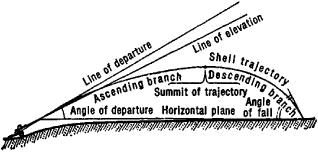Figure 1. Elements of a trajectory

Shells move along ballistic trajectories (Figure 1). In the case of small angles of fall (up to 20°), we speak of shallow trajectories and very low-angle fire; for angles of fall greater than 20°, we speak of steep trajectories and high-angle fire. When fired at airborne targets, antiaircraft shells—unlike artillery shells—have trajectories with only an ascending branch; the trajectories of rocket shells and rocket-assisted projectiles (for example, antitank rockets) have one or more powered phases, in which the rocket engines operate, and several free-flight phases. When the total length of the powered phases is small compared with the whole trajectory, the trajectory differs little from a ballistic trajectory; if the flight is controlled over the entire length of the trajectory or a substantial part of it, the trajectory differs considerably from a ballistic one.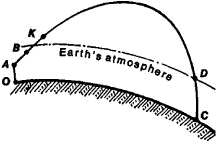Figure 2. The trajectory of a ballistic missile: (OA) launch phase, (AB) insertion phase, (BK) guided phase, (KD) segment outside the atmosphere, (DC) atmospheric phase

The trajectories of ballistic missiles have powered and free-flight phases (Figure 2). In the powered phase of a trajectory the specified speed and tilt angle that a ballistic missile should have at the end of this phase are imparted to the missile. The free-flight phase consists of two segments, a segment outside the atmosphere, in which the missile’s nose cone moves like a freely thrown body, and an atmospheric segment, in which the nose cone is stabilized and approaches the target nose first.

### REFERENCES

Dmitrievskii, A. A. Vneshnaia ballistika. Moscow, 1972.
Dmitrievskii, A. A. Fizicheskie osnovy poleta raket. Moscow, 1962. (With V. N. Koshev.)

A. A. LATUKHIN

## Trajectory

the continuous curve described by a point in motion. If the trajectory is a straight line, the motion of the point is said to be rectilinear; otherwise, it is said to be curvilinear. The shape of a trajectory of a free material particle depends on the forces acting on the particle, the initial conditions of the motion, and the reference frame used. For a constrained particle the trajectory depends also on the constraints that are imposed (seeCONSTRAINT).

For example, a vertical line is the trajectory with respect to the earth (if we neglect its diurnal rotation) of a free particle that is released without an initial velocity and moves under the influence of gravity. If, however, an initial velocity v0 not directed along the vertical is imparted to the particle, then, in the absence of air resistance, the trajectory is a parabola (Figure 1).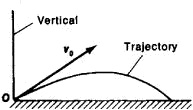Figure 1. A parabolic trajectory

Depending on the initial velocity, the trajectory of a particle moving in a central gravitational field may be an ellipse, a parabola, or a hyperbola; in special cases, the trajectory may be a straight line or a circle. If we regard the earth’s gravitational field as a central field and neglect atmospheric resistance, then the trajectory of a particle given a horizontally directed initial velocity v0 near the surface of the earth (Figure 2) is a circle when(orbital velocity), an ellipse when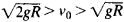, a parabola when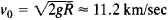(escape velocity), and a hyperbola when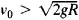. Here, R is the earth’s radius, g is the acceleration of gravity near the earth’s surface, and the motion is considered with respect to axes that are undergoing translational motion, together with the center of the earth, relative to the stars.

In the case of a body, such as a satellite, all the above remarks apply to the trajectory of the body’s center of gravity. If, however, the direction of v0 is neither horizontal nor vertical and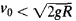, the trajectory of the particle is an arc of an ellipse; this arc intersects the earth’s surface. The center of gravity of a ballistic missile has such a trajectory.

An example of a constrained particle is a small bob suspended on a string (seePENDULUM). If the string is deflected from the vertical and released without an initial velocity, the trajectory of the bob is an arc of a circle. If, however, an initial velocity that does not lie in the plane of the string’s deflection is imparted to the bob, the trajectories of the bob may be curves of rather complicated shape that lie on the surface of a sphere; such trajectories are characteristic of a spherical pendulum. In the special case of a conical pendulum, the trajectory of the bob is a circle lying in the horizontal plane.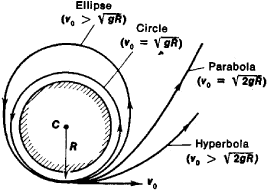Figure 2. Types of trajectories in the earth’s gravitational field

The trajectories of the points of a rigid body depend on the law of the body’s motion. For a translational motion of the body the trajectories of all its points are identical, but in all other cases the trajectories are generally different for the various points of the body. For example, for an automobile wheel on a rectilinear segment of a road, the trajectory of a point on the wheel’s rim relative to the highway is a cycloid, but the trajectory of the wheel’s center is a straight line. Relative to the automobile’s body, the trajectory of a point on the rim is a circle, but the wheel’s center is a fixed point.

The determination of trajectories is important both in theoretical studies and in the solution of many practical problems.

S. M. TARG

## trajectory

[trə′jek·trē]
(geophysics)
The path followed by a seismic wave.
(mathematics)
A curve that intersects all the members of a given family of curves at the same angle.
(mechanics)
The curve described by an object moving through space, as of a meteor through the atmosphere, a planet around the sun, a projectile fired from a gun, or a rocket in flight.
References in periodicals archive ?
Caption: Figure 10: Time history of mass of spacecraft in return trajectory.
Caption: Figure 11: Time history of attack angle rate a of spacecraft in return trajectory.
The expectations of Paul, the other representative of the High Match trajectory, were also formed from the comments filled with pride that he heard from the employees of the organization.
It is worth mentioning that the epithet "unfaithful" in "unfaithful sentinel" is of little significance in trajectory A but very important to trajectory B.
A first observation would be that the trajectory of the ball depends on three initial parameters: the launching speed, the angular velocity [omega] and the throw angle [alpha] being determined uniquely by them, the rest being constant (ball mass, radius, throw height h and distance horizontally to the edge of the semicircle D).
In doing so, we take into account that a firm's particular international trajectory is liable to affect and be affected by the firm's other international trajectories (Nachum & Song, 2011).
In order to better describe the algorithm proposed, we first need to describe the trajectory and its related properties.
Because the tracking algorithm based on model predictive control for the forecast of the future trajectory and the ability to deal with multi-objective constraints is strong, so it gradually becomes the hot research spot.
This burning is essential to offload resources and set the correct trajectory, and the precise duration of engine burn is set to 4 minutes and 45 seconds.
This paper is organized as follows: entry dynamics including entry trajectory constraints and vehicle model are described in Section 2; three-dimensional reentry trajectory-oscillation suppressing scheme is presented in Section 3; steady glide dynamic modeling and trajectory optimization are presented in detail in Section 4.
The dense trajectory proposed by  has a good result, but the method of refining the trajectories is still not perfect.

Site: Follow: Share:
Open / Close